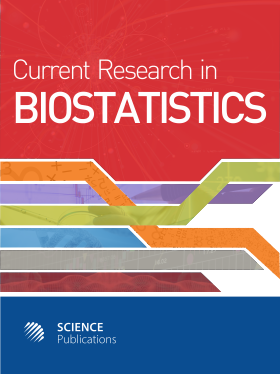Frequency: Continuous
ISSN: 2524-2210 (Print)
ISSN: 2524-2229 (Online)
Research Article Open Access

# Design Based on Intra-Class Correlation Coefficients

Robert Lew1 and Gheorghe Doros1
• 1 Boston University, United States

## Abstract

Problem statement: Reliability studies are concerned with the study of "consistency" or "repeatability" of measurements. Often times (but not always) the reliability coefficients are Intra-class Correlation Coefficients (ICC). Depending on the design or the conceptual intent of the study there are three types of intra-class correlation coefficients, termed intra-class correlation coefficients Case 1, 2 and 3, for measuring the reliability of a single interval measure. While methods for sample size calculations for intra-class correlation coefficients in Case 1 are available and implemented in Power Analysis and Sample Size System (PASS); to our knowledge, no methods based on intra-class correlation coefficients in Case 2 and 3 are available. Objective: Develop a method for calculating the size of a reliability study based on intra-class correlation coefficients Case 1 and 2. Approach: A practical method for computing sample size using simulations was proposed. We proposed to compute sample size based on the expected width of the confidence interval. For a given target value of the intra-class correlation coefficient, the proposed method chooses the design assures a 95% confidence interval with average length shorter than a pre-specified value. The applicability of the proposed method in practice for intra-class coefficients Case 2 was supported by demonstrating three invariance properties of the proposed confidence intervals. Results: Tables with sample size requirements were derived and displayed. A program for carrying out the calculations was developed in R. The method was used to size a trial aimed to study the reliability of a scale that measures the cleanness of the colon at the time of colonoscopy. Conclusion: A simple method for sample size calculation for intra-class correlation coefficient Case 1 and 2 based on the average length of confidence intervals was proposed. The proposed was implemented by the authors in R (freely available software). Three invariance properties of the confidence intervals for the intra-class correlation coefficients Case 2 were studied by simulations. These properties are an important tool when considering the design of this type of studies.

Current Research in Biostatistics
Volume 1 No. 1, 2010, 1-8

DOI:

Submitted On: 18 July 2009 Published On: 30 June 2010

How to Cite: Lew, R. & Doros, G. (2010). Design Based on Intra-Class Correlation Coefficients. Current Research in Biostatistics, 1(1), 1-8. https://doi.org/10.3844/amjbsp.2010.1.8

• 4,492 Views